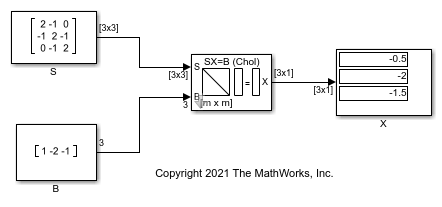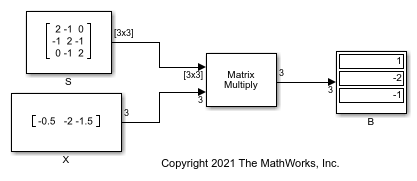# Solve Matrix Equation Using Cholesky Solver

Solve a matrix equation using the Cholesky Solver block.

Open and run the model. The model solves the equation SX = B using the Cholesky Solver block. The block uses the S and B matrices as inputs and outputs the solution matrix X. Matrix S must be a positive definite matrix.You can verify the solution by using the Matrix Multiply block to perform the multiplication SX, as shown in the following model. The output matrix B in this model equals the input matrix B in the previous model.